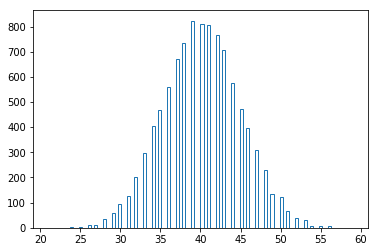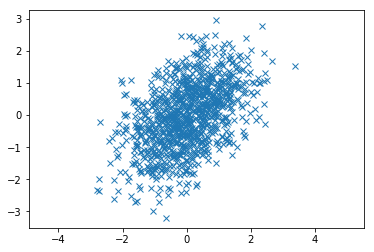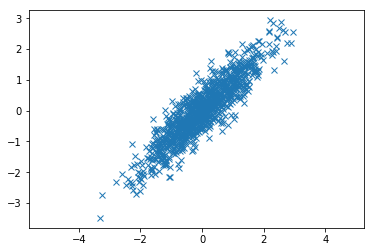### 生成特定分布的连续数据

#### 正态分布#### 二项分布### 生成独立的双变量数据

array([[ 1. , -0.00209488],
[-0.00209488, 1. ]])

### 生成相关为0.5的双变量数据

$$Corr(X, Y) = {Cov(X, Y) \over {(Var(X)Var(Y))^{0.5}}}$$array([[1. , 0.47665845],
[0.47665845, 1. ]])### 挑战: 按照因子载荷生成数据

$F$就是因子矩阵, 通常我们称为m个潜变量, 潜变量之间是正交的, 也就是相关为0, $\mu$ 是X的均值, 所以可以假设为0, 假设$X = [X_1, X_2, X_3]$, 则$\mu = [0, 0, 0]$, $epsilon$是特殊因子向量, 也就是不能被公共因子F所解释的, 并且与$\epsilon$

(1000, 3)

$$X_1 = 0.8*F_1 + 0.1*F_2 + 0.1*F_3 X_2 = 0.2*F_1 + 0.7*F_2 + 0.1*F_3 X_3 = 0.2*F_1 + 0.3*F_2 + 0.5*F_3$$

array([[0.8, 0.2, 0.2],
[0.1, 0.7, 0.3],
[0.1, 0.1, 0.5]])

(1000, 3)

### 因子分析

array([[1. , 0.75914447, 0.81103438],
[0.75914447, 1. , 0.88279048],
[0.81103438, 0.88279048, 1. ]])

array([2.63636942, 0.25335172, 0.11027886])

$$A = (...,\lambda_i^{0.5} l_i, ...)$$

array([[ 0.91181616, 0.40600843, -0.06122448],
[ 0.9405524 , -0.27299534, -0.20207603],
[ 0.95933408, -0.11824727, 0.25631172]])

### 赞助